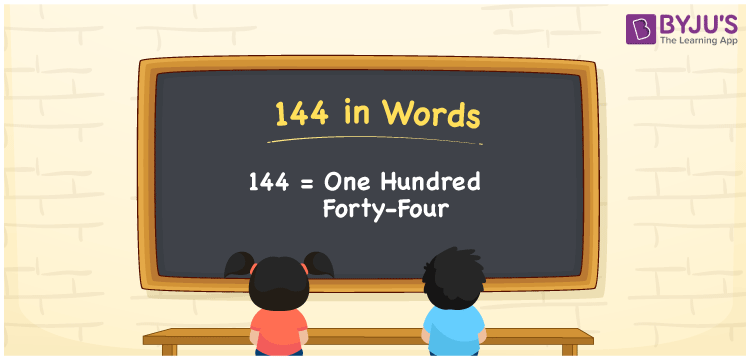# 144 in Words

We know that the number name is used to communicate, spell, or tell the same to others. For example, the number name of 9 is nine, which is used to spell the number 9. Similarly, the number name of 144 is One hundred forty-four. Thus, we can write 144 in words as One hundred forty-four. In this article, you will learn how to write the number 144 in words using a place value chart in detail.

 144 in words One hundred forty-four One hundred forty-four in Numbers 144

## 144 in English Words

We generally express numbers in words using the English alphabet. Thus, we can spell 144 in English as “One hundred forty-four”.## How to Write 144 in Words?

Let’s learn how to convert the number 144 into words. For this, create a three-column chart to assign the place value for all three digits of 144. This can be done as shown below.

 Hundreds Tens Ones 1 4 4

Here, we can observe that, ones = 4, tens = 4, hundreds = 1

We can write the expanded form as:

1 × Hundred + 4 × Ten + 4 × One

= 1 × 100 + 4× 10 + 4 × 1

= 100 + 40 + 4

= One hundred + Forty + Four

= One hundred forty-four

Therefore, 144 in words = One hundred forty-four

144 is a natural number that precedes 145 and succeeds 143.

144 in words – One hundred forty-four

Is 144 an odd number? – No

Is 144 an even number? – Yes

Is 144 a prime number? – No

Is 144 a composite number? – Yes

Is 144 a perfect square number? – Yes

What is the square root of 144? – 12

Is 144 a perfect cube number? – No

## Frequently Asked Questions on 144 in Words

Q1

### How do you write 144 in words?

The number 144 can be written as One hundred forty-four in words.
Q2

### How do you say 144 in English?

The spelling of 144 in English words is “One hundred forty-four”. Thus, we can spell the number 144 as One hundred forty-four.
Q3

### What number is One hundred forty-four in words?

One hundred forty-four is word form or the number name of 144.